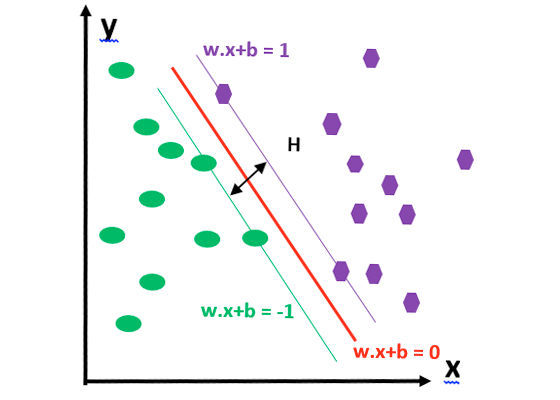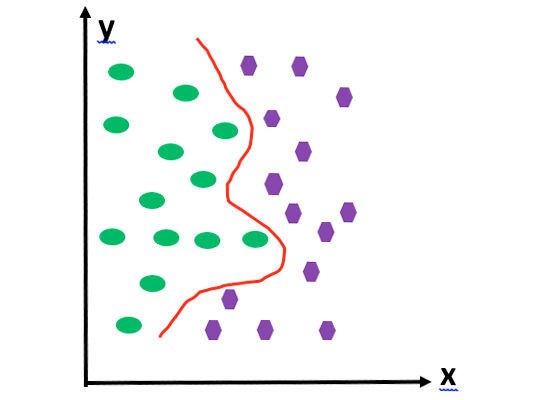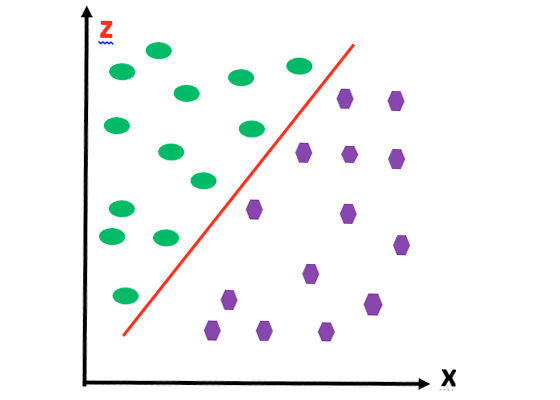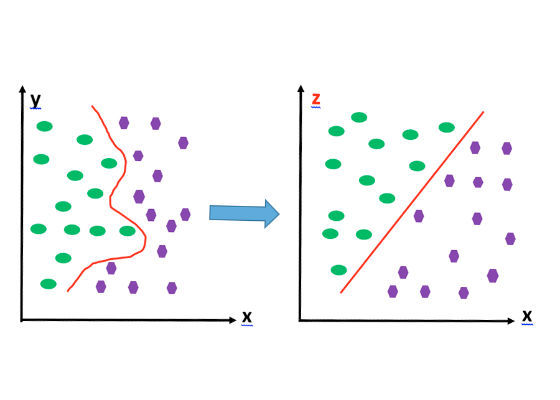# Improving Support Vector Machine with Intel Data Analytics Acceleration Library

By Promotion | Published on 31 May 2017Improving the Performance of Support Vector Machine with Intel® Data Analytics Acceleration Library

Introduction

With the wide availability of the internet, text categorization has become an important way to handle and organize text data. Text categorization is used to classify news stories and find information on the Web. Also, in order to search for a photo on the web or be able to distinguish a horse from a lion, for example, there must be some kind of mechanism to recognize and classify the pictures. Classifying text or pictures is time consuming. This type of classification is a good candidate for machine learning. 

This article describes a classification machine learning algorithm called support vector machine  and how the Intel® Data Analytics Acceleration Library (Intel® DAAL)  helps optimize this algorithm when running it on systems equipped with Intel® Xeon® processors.

What is a Support Vector Machine?

A support vector machine (SVM) is a supervised machine learning algorithm. It can be used for classification and regression.

An SVM performs classification by finding the hyperplane  that separates between a set of objects that have different classes. This hyperplane is chosen in such a way that maximizes the margin between the two classes to reduce noise and increase the accuracy of the results. The vectors that are on the margins are called support vectors. Support vectors are data points that lie on the margin.

Figure 1 shows how an SVM classifies objects:Figure 1:Classifying objects with a support vector machine.

There are two classes: green and purple. The hyperplane separates the two classes. If an object lies on the left side of the hyperplane, it is classified as belonging to the green class. Similarly, an object lying on the right side of the hyperplane belongs to the purple class.

As mentioned above, we need to maximize the margin H (the distance between the two margins) to reduce noise, thus improving the accuracy of the prediction.In order to maximize the margin H, we need to minimize |W|.

We also need to make sure that there are no data points lying between the two margins. To do that, the following conditions need to be met:

xi •w+b ≥ +1 when yi =+1
xi •w+b ≤ –1 when yi =–1
The above conditions can be rewritten to:
yi (xi •w) ≥ 1

So far we have talked about the hyperplane as being a flat plane or as a line in a two-dimensional space. However, in real-life situations, that is not always the case. Most of the time, the hyperplane will be curved, not straight, as shown in Figure 2.Figure 2:The hyperplane as a curved line.

For simplicity, assume that we are working in a two-dimensional space. In this case, the hyperplane is a curved line. To transform the curved line into a straight line, we can raise the whole thing into higher dimensions. How about lifting into a three-dimensional space by introducing a third dimension, called z?Figure 3:Introducing a third dimension, z.

The technique of raising the data to a higher dimensional space so that we can create a straight line or a flat plane in a higher dimension is called a kernel trick .Figure 4:Using a kernel trick to create a straight line or flat plane in a higher dimension.

Applications of SVM

SVMs can be used to:

• Categorize text and hypertext.
• Classify images.
• Recognize hand-written characters.

• Works well with a clear margin of separation.
• Effective in high-dimensional spaces.
• Uses a subset of training points in the decision function (called support vectors), so it is also memory efficient.

• Takes a huge amount of time to train a large dataset.
• Doesn’t work well when there is no clear separation between target classes.

Intel® Data Analytics Acceleration Library

Intel DAAL is a library consisting of many basic building blocks that are optimized for data analytics and machine learning. These basic building blocks are highly optimized for the latest features of latest Intel® processors. More about Intel DAAL can be found at . The SVM classifier is one of the classification algorithms that Intel DAAL provides. In this article, we use the Python* API of Intel DAAL, to build a basic SVM classifier. To install it, follow the instructions in .

Using Support Vector Machine Algorithm in Intel Data Analytics Acceleration Library

This section shows how to invoke the SVM algorithm  in Python  using Intel DAAL.

Do the following steps to invoke the SVM algorithm from Intel DAAL:

1. Import the necessary packages using the commands from and import

1. Import Numpy by issuing the command:

import numpy as np

2. Import the Intel DAAL numeric table by issuing the following command:

from daal.data_management import HomogenNumericTable

3. Import the SVM algorithm using the following commands:

from daal.algorithms.svm import training, prediction
from daal.algorithms import classifier, kernel_function
import daal.algorithms.kernel_function.linear

2. Create a function to split the input dataset into the training data, label, and test data.

Basically, split the input data set array into two arrays. For example, for a dataset with 100 rows, split it into 80/20: 80 percent of the data for training and 20 percent for testing. The training data will contain the first 80 lines of the array input dataset, and the testing data will contain the remaining 20 lines of the input dataset.

3. Restructure the training and testing dataset so Intel DAAL can read it.

Use the commands to reformat the data as follows (We treat trainLabels and testLabels as n-by-1 tables, where n is the number of lines in the corresponding datasets):

trainInput = HomogenNumericTable(trainingData)
trainLabels = HomogenNumericTable(trainGroundTruth.reshape(trainGroundTruth.shape,1))
testInput = HomogenNumericTable(testingData)
testLabels = HomogenNumericTable(testGroundTruth.reshape(testGroundTruth.shape,1))
where
trainInput: Training data has been reformatted to Intel DAAL numeric tables.
trainLabels: Training labels has been reformatted to Intel DAAL numeric tables.
testInput: Testing data has been reformatted to Intel DAAL numeric tables.
testLabels: Testing labels has been reformatted to Intel DAAL numeric tables.
Create a function to train the model.

First create an algorithm object to train the model using the following command:
algorithm = training.Batch_Float64DefaultDense(nClasses)

Pass the training data and label to the algorithm using the following commands:
algorithm.input.set(classifier.training.data,trainInput)
algorithm.input.set(classifier.training.labels,trainLabels)
where
algorithm: The algorithm object as defined in step a above.
trainInput: Training data.
trainLabels: Training labels.
Train the model using the following command:
Model = algorithm.compute()
where
algorithm:The algorithm object as defined in step a above.

4. Create a function to test the model.

1. First create an algorithm object to test/predict the model using the following command:

algorithm = prediction.Batch_Float64DefaultDense(nClasses)

2. Pass the testing data and the train model to the model using the following commands:

algorithm.input.setTable(classifier.prediction.data, testInput) algorithm.input.setModel(classifier.prediction.model, model.get(classifier.training.model))
where
algorithm: The algorithm object as defined in step a above.
testInput: Testing data.
model: Name of the model object.

trainLabels: Training labels.

3. Train the model using the following command:

Model = algorithm.compute()
where
algorithm:The algorithm object as defined in step a above.

5. Create a function to test the model.

1. First create an algorithm object to test/predict the model using the following command:
algorithm = prediction.Batch_Float64DefaultDense(nClasses)

2. Pass the testing data and the train model to the model using the following commands:

algorithm.input.setTable(classifier.prediction.data, testInput) algorithm.input.setModel(classifier.prediction.model, model.get(classifier.training.model))
where
algorithm: The algorithm object as defined in step a above.
testInput: Testing data.
model: Name of the model object.

3. Test/predict the model using the following command: Prediction = algorithm.compute()

where
algorithm: The algorithm object as defined in step a above.
prediction: Prediction result that contains predicted labels for test data.

Conclusion

SVM is a powerful classification algorithm. It works well with a clear margin of separation. Intel DAAL optimized the SVM algorithm. By using Intel DAAL, developers can take advantage of new features in future generations of Intel® Xeon® processors without having to modify their applications. They only need to link their applications to the latest version of Intel DAAL.

For more such intel IoT resources and tools from Intel, please visit the Intel® Developer Zone

Source:https://software.intel.com/en-us/articles/improving-svm-performance-with-intel-daal### Popular Mobile Phones

View All

hot deals

View All

Digit caters to the largest community of tech buyers, users and enthusiasts in India. The all new Digit in continues the legacy of Thinkdigit.com as one of the largest portals in India committed to technology users and buyers. Digit is also one of the most trusted names when it comes to technology reviews and buying advice and is home to the Digit Test Lab, India's most proficient center for testing and reviewing technology products.